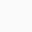### 3 Concepts Every Data Scientist Must Know Part – 2

•• 1

## 1. Bagging and Boosting

Bagging and Boosting are two different ways used in combining base estimators for ensemble learning (Like random forest combining decision trees). Bagging means aggregating the predictions of several weak learners. We can think of it combining weak learners is used in parallel. The average of the predictions of several weak learners is used as the overall prediction.

Boosting means combing several weak learners in series. We end up having a strong learner from many sequentially connected weak learners. One of most commonly used ensemble algorithm that uses boosting is gradient boosted decision tree (GBDT). Like in random forests, weak learners (or base estimators) in GBDT are decision trees.

## 2. Entropy and Information Gain

Entropy is a measure of uncertainty or randomness. The more randomness a variable has, the higher the entropy is. The variables with uniform distribution have the highest entropy. For example, rolling a fair dice has 6 possible outcomes with equal probabilities soo it has a uniform distribution and high entropy.

We are likely to come across entropy and information gain when dealing with decision trees. They are the determining factors when the algorithm decides on splits. Splits that result in more pure nodes are chosen. All these indicate “information gain” which is basically the difference between entropy before and after the split.

When choosing a feature to split, decision tree algorithm tries to achieve

More predictiveness
Less impurity
Lower entropy

## 3. Precision and Recall

Precision and recall are metrics used to evaluate classification models. Before describing these metrics, it is better to explain the confusion matrix. Confusion matrix shows the correct and incorrect (i.e. true or false) predictions on each class. In class of a binary classification task, a confusion matrix is a 2×2 matrix. If there are three different classes, it is a 3×3 matrix and so on.

Let’s assume class A is positive class and class B is negative class.

The key terms of confusion matrix are as follows:

True positive (TP): Predicting positive class as positive (ok).

False positive (FP): Predicting negative class as positive (not ok).

False negative (FN): Predicting positive class as negative (not ok).

True negative (TN): Predicting negative class as negative (ok).

Precision indicates how many positive predictions are correct. The focus of precision is positive predictions.

Recall indicates how many of the positive classes the model is able to predict correctly. The focus is actual positive classes.

## Popular Posts

1.meteorite says: